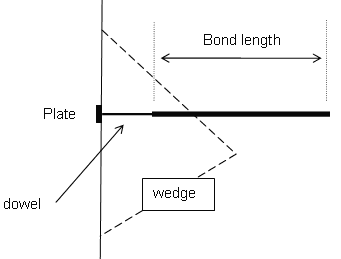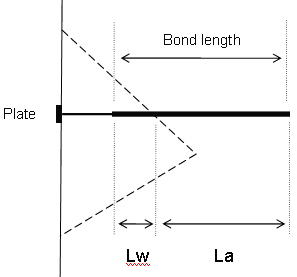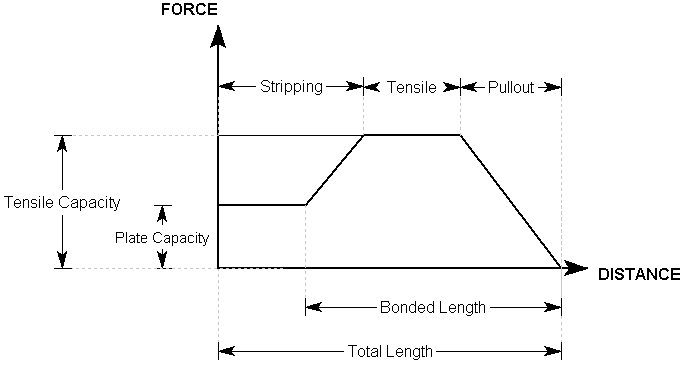The Rocscience International Conference 2021 Proceedings are now available. Read Now

# Grouted Dowel

The Grouted Dowel bolt type can be used to model support which has a variable bonded (grouted) length.Grouted dowel with bonded length and face plate

## Tensile Capacity

The Tensile Capacity represents the maximum tensile capacity of the bolt itself (i.e., steel tensile capacity), independent of the plate capacity or the bond capacity. Units are Force.

## Plate Capacity

The Plate Capacity is the maximum load which can be sustained by the plate assembly which connects the dowel to the exposed wedge face. Units are Force.

## Bond Strength

The Bond Strength is expressed as a Force per unit Length, where the length refers to the length along the dowel. The maximum pullout force is therefore simply the Bond Length of the dowel, multiplied by the Bond Strength.

## Bond Length

The bonded (grouted) length can be specified as either:

• A percentage of length
• Actual bond length

NOTE: The bonded length is always measured from the end of the bolt, and is displayed on the model as a thicker line drawn over each bolt.

## Bolt Force Diagram

The Grouted Dowel tensile properties which are entered in the Bolt Properties dialog, are used to determine a Force Diagram for the dowel, as described below.

Consider a dowel which intersects a wedge as follows.Lw = wedge length = bonded length of dowel within wedge

La = anchorage length = bonded length of dowel embedded in rock beyond wedge

Dowel Parameters:

T = Tensile Capacity (force)

P = Plate Capacity (force)

B = Bond Strength (force / unit length of bond)

For a Grouted Dowel, at any point along the length of the dowel, there are 3 possible failure modes which are considered:

1. Pullout (force required to pull the anchorage length La of the bolt out of the rock)
2. Tensile Failure (maximum axial capacity of the bolt)
3. Stripping (wedge failure occurs, and bolt remains embedded in rock)

The maximum force which can be mobilized by each failure mode, at any point along the bolt length, is given by the following equations:

Pullout: F1 = BLa Eqn.1

Tensile: F2 = T Eqn.2

Stripping: F3 = P + BLw Eqn.3

NOTE: In order for stripping to occur, the Plate Capacity must be exceeded. The Plate Capacity is included in the stripping force equation, and added to the bond capacity along the wedge length Lw.

At any point along the length of the bolt, the force which is applied to the wedge by the bolt, is given by the MINIMUM of these three forces.

Applied Force = min (F1, F2, F3) Eqn.4

A Force diagram for a Grouted Dowel, which exhibits all three failure modes, is shown below. In this case, the Plate Capacity is less than the Tensile Capacity, and therefore "stripping" is a possible failure mode. If the Plate Capacity is greater than the Tensile Capacity, then stripping cannot occur, and the Force diagram will be determined only by the Tensile and Pullout failure modes.Tensile bolt force diagram for grouted dowel

## Bolt Orientation Efficiency

The tensile capacity determined from the Bolt Force Diagram, assumes that a bolt is mobilized in pure tension. However, this will usually not be the case, and a bolt which intersects a wedge may also be in shear or even compression.

A Bolt Orientation Efficiency factor (0 to 1) can be applied to the tensile capacity of the bolt determined from the Bolt Force Diagram. The efficiency factor accounts for the fact a bolt will in general not be mobilized in pure tension, but may include shear or compression. See the Bolt Orientation Efficiency topic for more information.

## Shear Strength

A bolt may also support a wedge by virtue of the shear strength of the bolt, acting against the sliding direction of the wedge. Shear strength of a bolt may be considered in the analysis by selecting the Use Shear Strength checkbox, and entering a value of Shear Strength.

NOTE: For any given bolt, only one support force will ultimately be applied, Shear or Tensile. Both modes cannot operate simultaneously. For more information, see the Bolt Shear Strength topic and the Bolt Support Force topic, for an overview of the support force implementation in UnWedge.

## Display of Bolt Force Diagrams

The Bolt Force Diagram for each bolt can be displayed directly on the model, as a display option. See the Bolt Force Diagrams topic for more information.